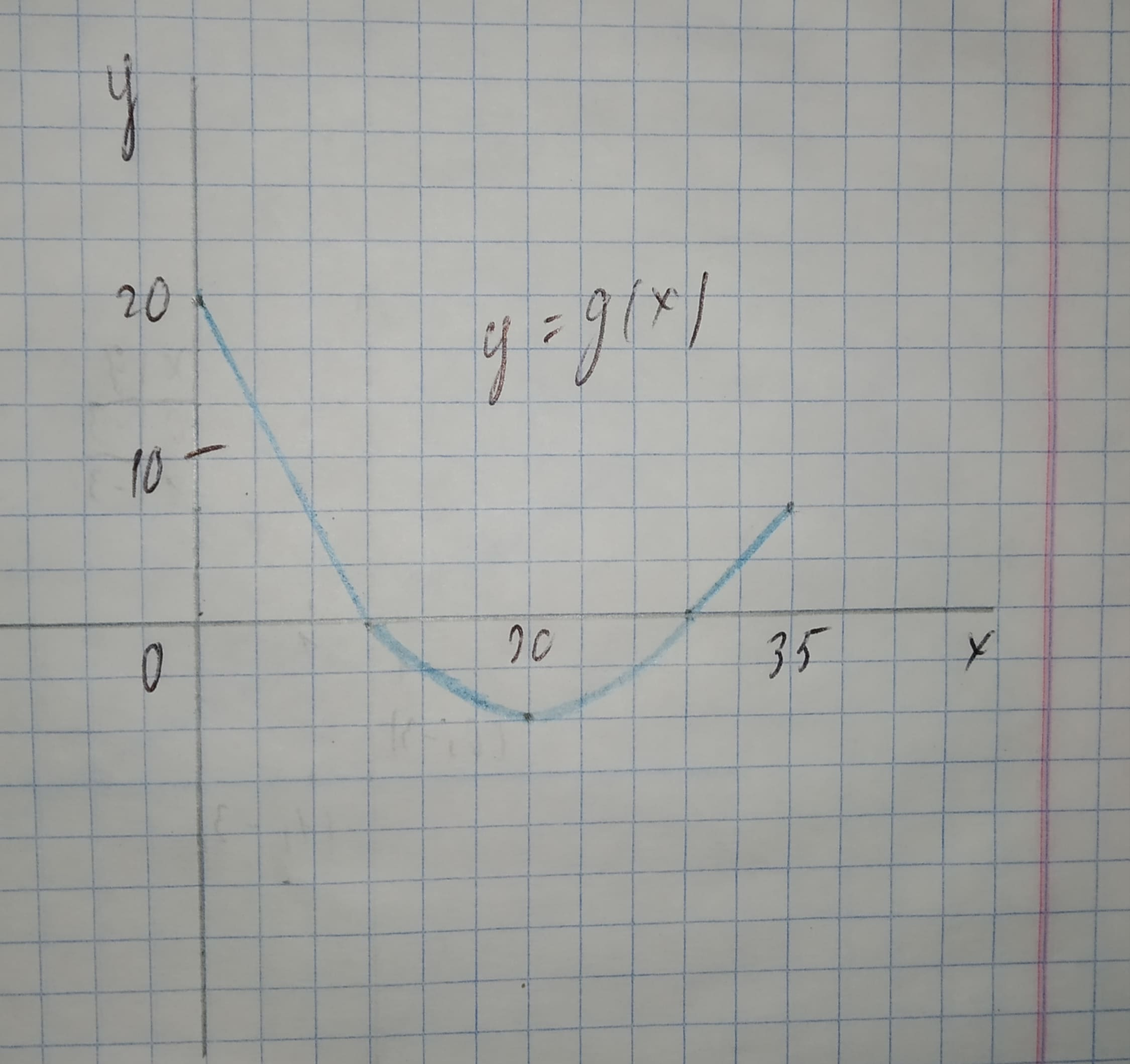# The graph of g consists of two straight lines and a semicircle. Use it to eveluate the integral.12210203861.jpgint_0^10 g(x)dxsanuluy 2021-02-01 Answered

The graph of g consists of two straight lines and a semicircle. Use it to eveluate the integral.${\int }_{0}^{10}g\left(x\right)dx$

You can still ask an expert for help

• Questions are typically answered in as fast as 30 minutes

Solve your problem for the price of one coffee

• Math expert for every subject
• Pay only if we can solve itodgovoreh
The integral ${\int }_{0}^{10}g\left(x\right)dx$ is the area between the graph of g and the horizontal x-axis on the interval $0\le x\le 10$.
We note that this are forms a triangle with base b = 10 and height h = 20.
The area of a triangle is the product of the base b and the height h, divided by 2.
${\int }_{0}^{10}g\left(x\right)dx=\frac{b\cdot h}{2}$
$=\frac{10\cdot 20}{2}$
$=\frac{200}{2}$
=100